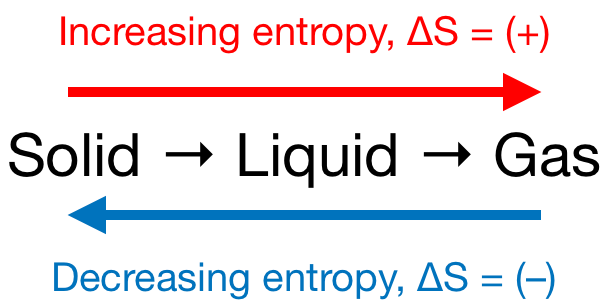# Problem: Which of the following processes have a ΔS &gt; 0?a. 2NH3(g) + CO2(g) → NH2CONH2(aq) + H2O(l)b. lithium fluoride forms from its elementsc. 2HF(g) → H2(g) + F2(l)d. potassium iodide dissolves in pure water

###### FREE Expert Solution

We’re asked to determine which of the following processes have a ΔS > 0.

Also, recall that if:

• ΔS > 0 or ΔS = (+); entropy is increased

• ΔS < 0 or ΔS = (–); entropy is decreased

Remember that phase changes lead to a change in entropy:Also, recall that:

• An increase in the number of gas molecules in the reaction also leads to an increase in entropy.
• If bonds are broken then the entropy of a reaction increases,
• But, if bonds are formed then the entropy of a reaction decreases.

99% (299 ratings)###### Problem Details

Which of the following processes have a ΔS > 0?

a. 2NH3(g) + CO2(g) → NH2CONH2(aq) + H2O(l)

b. lithium fluoride forms from its elements

c. 2HF(g) → H2(g) + F2(l)

d. potassium iodide dissolves in pure water